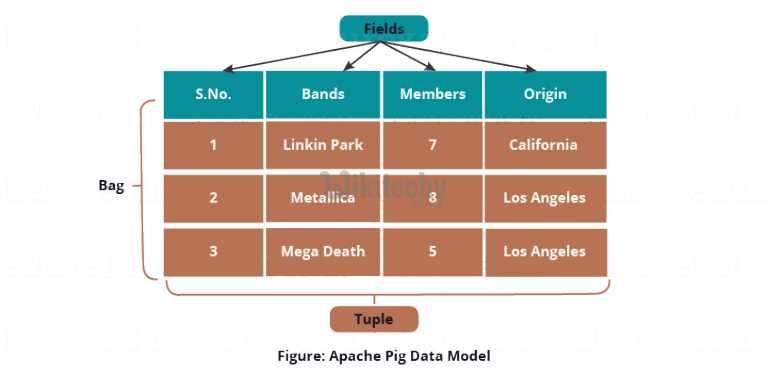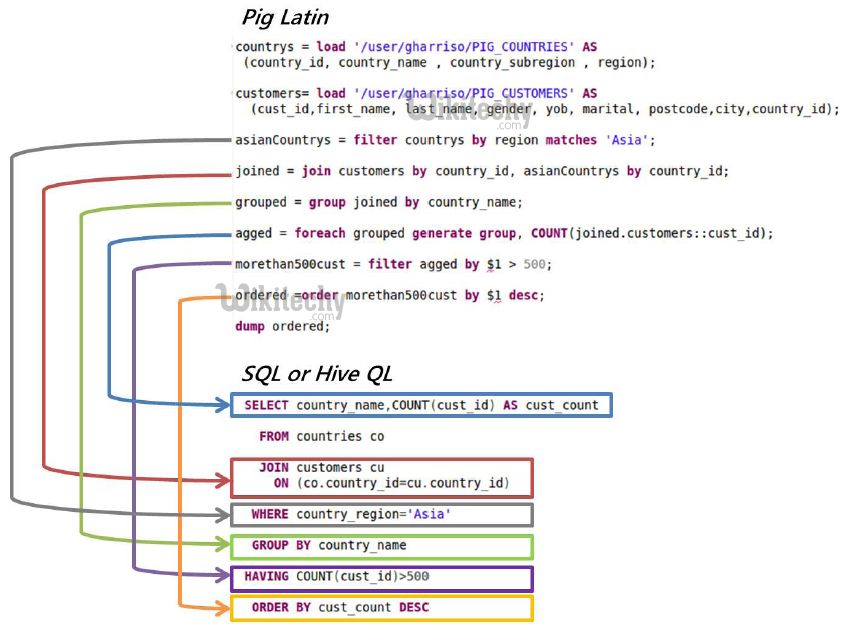# pig tutorial - apache pig tutorial - Apache Pig Latin - Basics - pig latin - apache pig - pig hadoop

## What is Pig Latin?

• The Pig Latin basics are given as Pig Latin Statements, data types, general and relational operators, and Pig Latin UDF’s.
• Pig Latin is the language which is used to analyze data in Hadoop by using Apache Pig.
• Pig Latin is a dataflow language where each processing step will result in a new data set, or in a relation.

## Pig Latin - Data Model

• The data model of Pig Latin is fully nested. The Relation in Pig Latin is the structure of the Pig Latin data model. And it has a bag where −
• A bag has a collection of tuples.
• A tuple is an ordered set of fields which is given.
• A field is the piece of data.Learn apache pig - apache pig tutorial - Apache Pig Latin Basics - apache pig examples - apache pig programs

## Pig Latin vs hiveql• The data model of Pig Latin is fully nested.
• The Relation in Pig Latin is the structure of the Pig Latin data model. And it has a bag where −
• Pig Latin Statements are the basic statements while processing the data by using Pig Latin.
• The statements work with relations used in Pig Latin and they include the expressions and schemas.
• The every statement which is given in Pig Latin will end with a semicolon (;).
• We can perform various operations by using operators which is provided by Pig Latin done through statements.
• Pig Latin statements take a relation as input and produce another relation as output except for Load and Store while performing the other operations in Pig Latin
• When we enter a Load statement in the Grunt shell, the semantic checking for Load store will be carried out.
• If we need to see the contents of the schema, we can use the Dump operator.
• The MapReduce job is done for loading the data which is done into the file system will be carried out only after performing the dump operation which is done in Pig Latin.

## Pig Latin - Data types

• The table which is given below describes the Pig Latin data types.

## Simple Types

Operator Description Example
intSigned 32-bit integer10
longSigned 64-bit integerData: 10L or 10l Display: 10L
float32-bit floating pointData: 10.5F or 10.5f or 10.5e2f or 10.5E2F Display: 10.5F or 1050.0F
double64-bit floating pointData: 10.5 or 10.5e2 or 10.5E2 Display: 10.5 or 1050.0
chararrayCharacter array (string) in Unicode UTF-8 formathello world
bytearrayByte array (blob)
booleanbooleantrue/false (case insensitive)

## Complex Types

Operator Description Example
tupleAn ordered set of fields.(19,2)
bagAn collection of tuples.{(19,2), (18,1)}
mapA set of key value pairs.[name#John,phone#5551212]

## Null Values

• The values for all the above data types which is given in a table can be NULL.
• Pig Latin treats null values in a same way as Apache Pig and SQL
• The null value which is given can be a non-existent value.
• The null value is used as a placeholder for optional values.
• The null value can be given as the result of an operation.

## Pig Latin - Arithmetic Operators

• The table which is given below describes the arithmetic operators of Pig Latin. For example consider a = 10 and b = 20.
Operator Description Example
+ Addition − Adds values on either side of the operator a + b will give 30
Subtraction − Subtracts right hand operand from left hand operand a − b will give −10
* Multiplication − Multiplies values on either side of the operator a * b will give 200
/ Division − Divides left hand operand by right hand operand b / a will give 2
% Modulus − Divides left hand operand by right hand operand
and returns remainder
b % a will give 0
? : Bincond − Evaluates the Boolean operators. It has three
operands as shown below.

variable x = (expression) ? value1 if true : value2 if false.

b = (a == 1)? 20: 30;

if a = 1 the value of b is 20.

if a!=1 the value of b is 30.

CASE

WHEN

THEN

ELSE END

Case − The case operator is equivalent to nested bincond operator. CASE f2 % 2

WHEN 0 THEN 'even'

WHEN 1 THEN 'odd'

END

## Pig Latin – Comparison Operators

The following table describes the comparison operators of Pig Latin.

Operator Description Example
==

Equal − Checks if the values of two operands are equal or not;
if yes, then the condition becomes true.

(a = b) is not true
!=

Not Equal − Checks if the values of two operands are equal or not.
If the values are not equal, then condition becomes true.

(a != b) is true.
>

Greater than − Checks if the value of the left operand is greater than
the value of the right operand. If yes, then the condition becomes true.

(a > b) is not true.
<

Less than − Checks if the value of the left operand is less than the
value of the right operand. If yes, then the condition becomes true.

(a < b) is true.
>=

Greater than or equal to − Checks if the value of the left operand is
greater than or equal to the value of the right operand. If yes, then the
condition becomes true.

(a >= b) is not true.
<=

Less than or equal to − Checks if the value of the left operand is
less than or equal to the value of the right operand. If yes, then the
condition becomes true.

(a <= b) is true.
matches

Pattern matching − Checks whether the string in the left-hand side
matches with the constant in the right-hand side.

f1 matches '.*tutorial.*'

## Pig Latin - Type Construction Operators

• The table which is given below describes the Type construction operators of Pig Latin.
Operator Description Example
()

Tuple constructor operator − This operator is used to construct a tuple.

(Raju, 30)
{}

Bag constructor operator − This operator is used to construct a bag.

[]

Map constructor operator − This operator is used to construct a tuple.

[name#Raja, age#30]

## Pig Latin - Relational Operations

• The table which is given below describes the relational operators of Pig Latin.
Operator Description
LOAD To Load the data from the file system (local/HDFS) into a relation.
STORE To save a relation to the file system (local/HDFS).
Filtering
FILTER To remove unwanted rows from a relation.
DISTINCT To remove duplicate rows from a relation.
FOREACH, GENERATE To generate data transformations based on columns of data.
STREAM To transform a relation using an external program.
Grouping and Joining
JOIN To join two or more relations.
COGROUP To group the data in two or more relations.
GROUP To group the data in a single relation.
CROSS To create the cross product of two or more relations.
Sorting
ORDER To arrange a relation in a sorted order based on one or more fields (ascending or descending).
LIMIT To get a limited number of tuples from a relation.
Combining and Splitting
UNION To combine two or more relations into a single relation.
SPLIT To split a single relation into two or more relations.
Diagnostic Operators
DUMP To print the contents of a relation on the console.
DESCRIBE To describe the schema of a relation.
EXPLAIN To view the logical, physical, or MapReduce execution plans to compute a relation.
ILLUSTRATE To view the step-by-step execution of a series of statements.